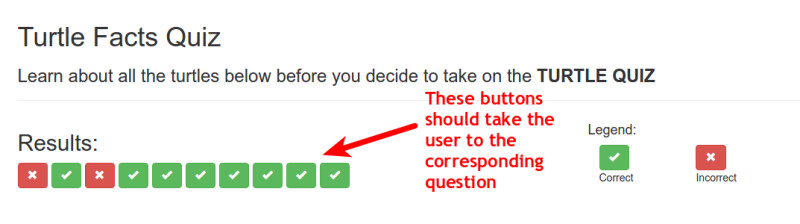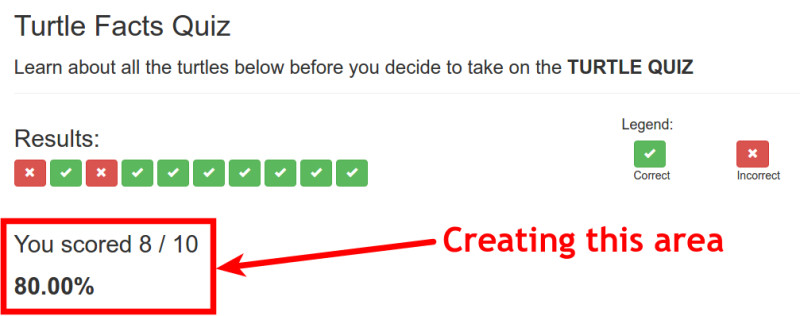# AngularJS Quiz App Tutorial Part 22 – Final Functions For RestultsCtrl

## Last Two Functions For The ResultsCtrl

In this part we will add the final two functions into the results controller. A function that will be similar to the setActiveQuestion in the quiz controller but not nearly as complex and the second function will be to calculate the percentage score the user got in the quiz. We will also briefly discuss another filter – the Angular number filter

If you want to see the app for yourself, check it out here

The git repo can be found here.

The next part can be found hereThe last thing we said in the last part was that clicking the buttons in the results area does nothing. So here we will create a function that allows the user to click any of those buttons and get taken to the corresponding question to see how they did.

So remember back to when we created the buttons in the results area, we added an ng-click that calls setActiveQuestion. But this of course, isn’t the same as the setActiveQuestion on the quiz controller, it just has the same name. We now need to create this new function.

In the ng-click we gave it an argument of \$index, so in the function we will need to take that argument and set the active question to that index. Remember \$index is coming from the ng-repeat. So if the user clicks on the 5th button from the left \$index will equal 4 (0 index remember), then the function sets the active question to 4, so we see the corresponding data displaying.

You may by now realise that we could get rid of this function and just add the code directly into the ng-click to change activeQuestion to \$index. If you did think that, you are perfectly correct and in fact, that way is probably better. I only used the function to expose everyone to as many ways of doing things as possible to really get an idea of how Angular works.

## Calculating User’s Percentage Score

The final area to add to the results page is the bit in between the buttons and the question which shows the percentage score the user got. In the HTML, between the buttons and question we will add the following HTML markup.Here we make use of the numCorrect property that we created earlier which is incremented in the markQuiz function everytime the user gets an answer correct. We also use the .length method on the quizQuestions object to find the total number of questions in the quiz.

The final thing you will notice is we call a function called calculatePerc. Which is what we will create now.

### A Small Caveat – Angular Number Filter

In this quiz we have 10 questions which will result in the percentage always being a nice whole number. But if you have a different number of questions, you may end up with a percentage that has many decimal places. This can look bad and you should keep that under control.

There is another Angular Filter that will allow you to filter the number down to a certain number of decimal places. This would be how to do that:>

The standard | is used to tell Angular we are going to use a filter and the filter we use is called number. The a colon followed by the arguments for the filter. In this case, the only argument is the number of decimal places that you want to display.

### Only Two Tasks Until We Have Finished The App

There are only two things left for us to do. The first is to fix the image questions again – just like we did in the quiz controller and the final task is to add the “go back to quiz” button. Then we are done.

In part 23 we will tackle fixing up the image questions.

See you there